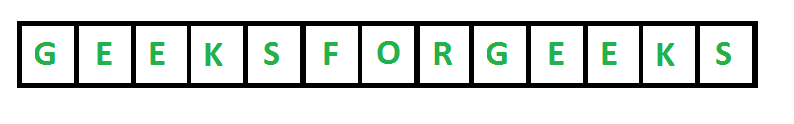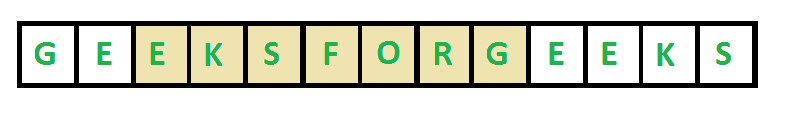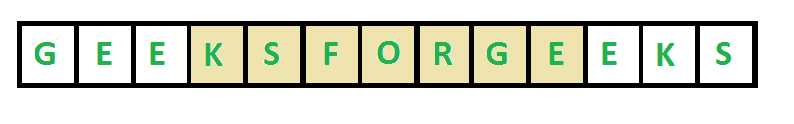Open In App

# Javascript Program To Find Length Of The Longest Substring Without Repeating Characters

Given a string str, find the length of the longest substring without repeating characters.

• For “ABDEFGABEF”, the longest substring are “BDEFGA” and “DEFGAB”, with length 6.
• For “BBBB” the longest substring is “B”, with length 1.
• For “GEEKSFORGEEKS”, there are two longest substrings shown in the below diagrams, with length 7The desired time complexity is O(n) where n is the length of the string.

Method 1 (Simple : O(n3)): We can consider all substrings one by one and check for each substring whether it contains all unique characters or not. There will be n*(n+1)/2 substrings. Whether a substring contains all unique characters or not can be checked in linear time by scanning it from left to right and keeping a map of visited characters. Time complexity of this solution would be O(n^3).

## Javascript

 ``

Output

```The input string is geeksforgeeks
The length of the longest non-repeating character substring is 7```

Method 2 (Better : O(n2)) The idea is to use window sliding. Whenever we see repetition, we remove the previous occurrence and slide the window.

## Javascript

 ``

Output

```The input string is geeksforgeeks
The length of the longest non-repeating character substring is 7```

Method 4 (Linear Time): Let us talk about the linear time solution now. This solution uses extra space to store the last indexes of already visited characters. The idea is to scan the string from left to right, keep track of the maximum length Non-Repeating Character Substring seen so far in res. When we traverse the string, to know the length of current window we need two indexes.
1) Ending index ( j ) : We consider current index as ending index.
2) Starting index ( i ) : It is same as previous window if current character was not present in the previous window. To check if the current character was present in the previous window or not, we store last index of every character in an array lasIndex[]. If lastIndex[str[j]] + 1 is more than previous start, then we updated the start index i. Else we keep same i.

Below is the implementation of the above approach :

## Javascript

 ``

Output

```The input string is geeksforgeeks
The length of the longest non-repeating character substring is 7```

Time Complexity: O(n + d) where n is length of the input string and d is number of characters in input string alphabet. For example, if string consists of lowercase English characters then value of d is 26.
Auxiliary Space: O(d)

Please refer complete article on Length of the longest substring without repeating characters for more details!